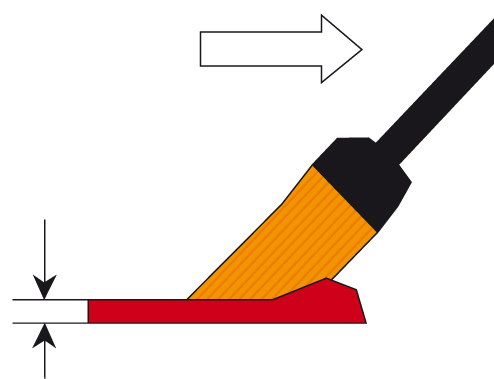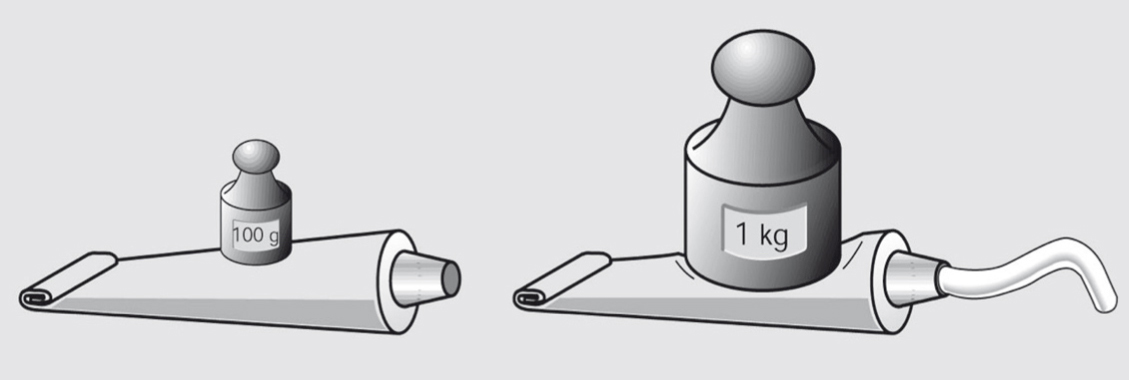44 Rates

# Examples of the calculation of shear rates

The following data is required for the shear rate calculation: A rheometer records the shear force via the torque at each measuring point. The torque is either preset or, if the rotational speed is the preset value, it is determined via the flow resistance force of the sample. The size of the shear area is also known for the measuring system used.

## Painting with a brush

Calculation of the shear rate using the two-plates model and the following definition:

$\dot \gamma = {v\over{h}}$

With a painting speed of v = 0.5 m/s and a thickness of the wet layer of h = 200 μm = 0.2 mm = 0.0002 m, the resulting shear rate is 2500 s-1Figure 1: Application of paint with a brush: Demonstration of calculating the shear rate based on the application velocity and the thickness of the wet paint layer.

## Flow of toothpaste out of a tube

The shear rate of flow in capillaries, tubes, and pipes is calculated using the Hagen/Poiseuille formula:

$\dot \gamma = {{(4 \cdot {V})}\over {p \cdot {R}^3\cdot {t}}}$

With a discharged volume of V = 1 cm3 and a flow time of t = 1 s, the volume flow rate is V/t = 10-6 m3/s. Based on a tube outlet with a diameter d = 6 mm or radius R = 3 • 10-3 m and circle constant pi = 3.14, the shear rate is:

$\dot \gamma ={{{(4\cdot{10^{-6}}{{m^3}\over{s}}})}\over{(pi \cdot {27} \cdot {10^{-9}}m^3)}}= 47.2 s^{-1}=approx. 50 s^{-1}$Figure 2: Squeezing toothpaste out of a tube: Demonstration of calculating the shear rate based on discharged volume, flow time, and radius of the tube’s nozzle.